May 10th, 2016

## Plan for Today

• Experimental Design
• Simulation

## Genome Assembly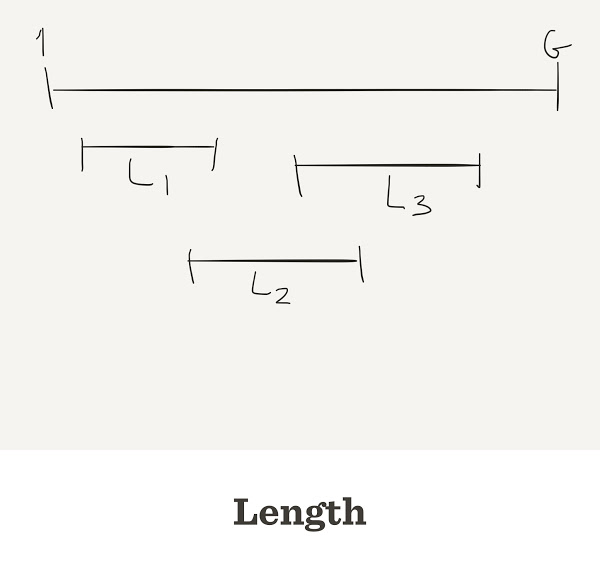## Genome Assembly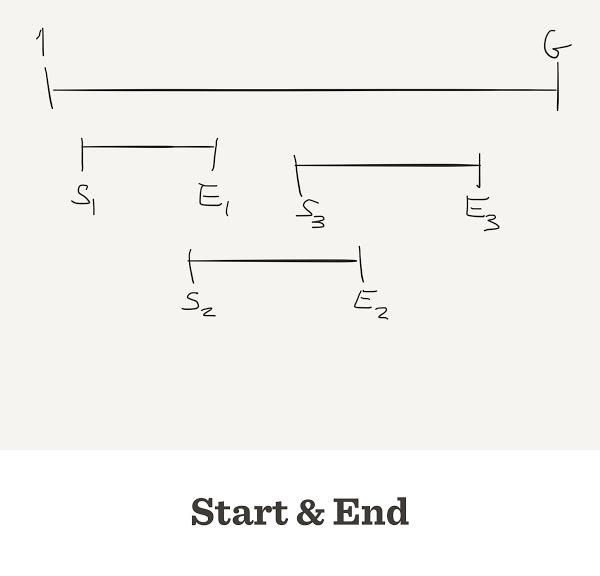## Genome Assembly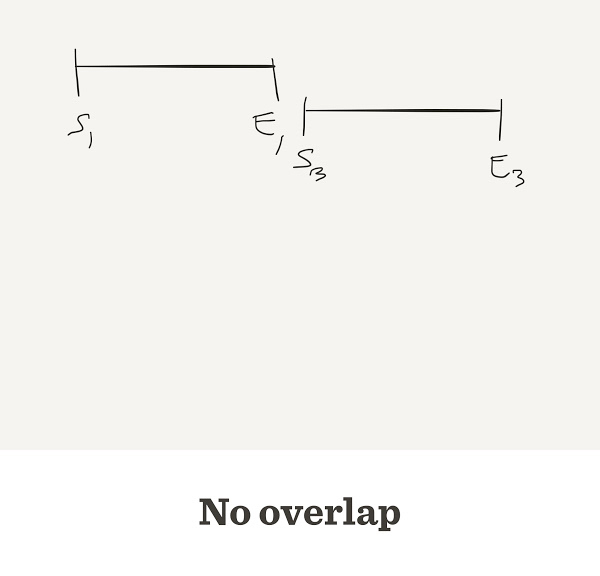## Genome Assembly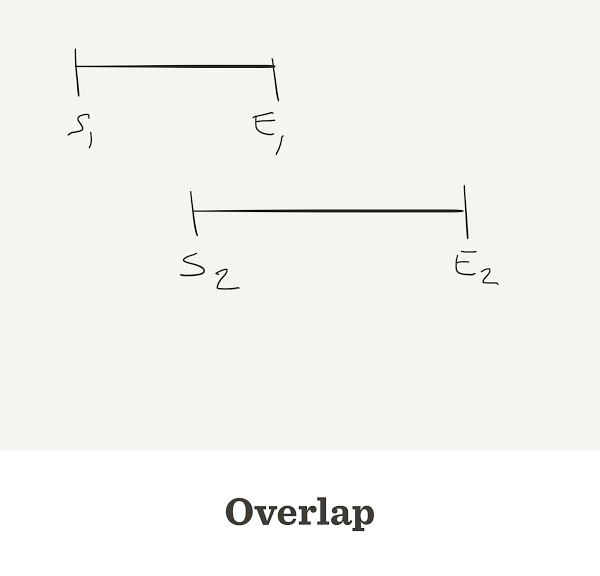## Genome Assembly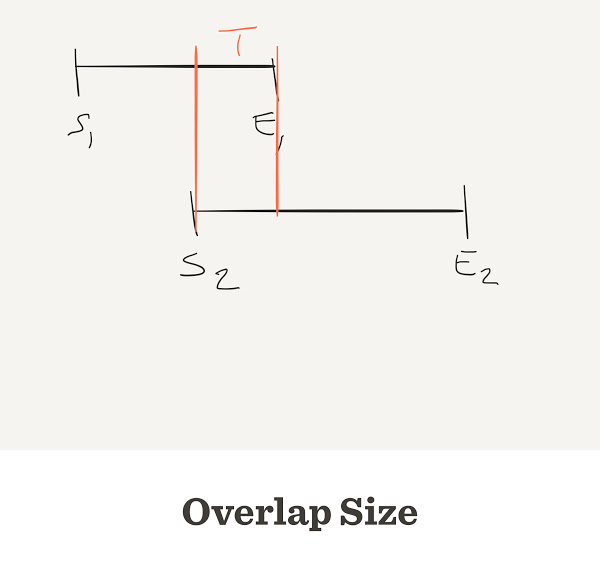## Gap counter

num.gaps <- function(G,L,n) {
start <- sample(G, n, replace=TRUE)
start <- sort(start)
end <- start + L
return(sum(start[-1] > end[-length(end)]))
}
• G: Length of genome

## Gap counter

num.gaps <- function(G,L,n) {
start <- sample(G, n, replace=TRUE)
start <- sort(start)
end <- start + L
gap <- start[-1] - end[-length(end)]
return(sum(gap > 0))
}

## Gap counter

num.gaps <- function(G,L,n) {
start <- sample(G, n, replace=TRUE)
start <- sort(start)
end <- start + L
overlap <- end[-length(end)] - start[-1]
return(sum(overlap < 0))
}

## Contig counter

num.gaps <- function(G,L,n,t) {
start <- sample(G, n, replace=TRUE)
start <- sort(start)
end <- start + L
overlap <- end[-length(end)] - start[-1]
return(sum(overlap < t))
}
• G: Length of genome